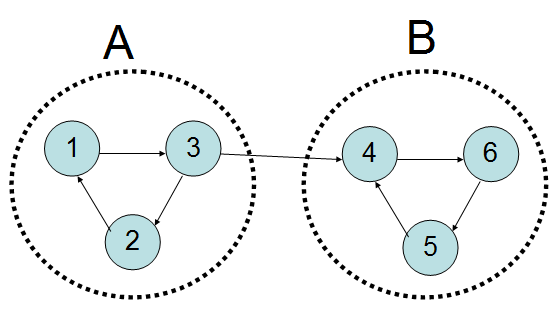# Luogu3387【模板】缩点（Kosaraju）

## 【模板】缩点

2 2
1 1
1 2
2 1

2

##### 说明

$n<=10^4,m<=10^5$,点权<=1000

##### 题解

1.在反向图上$dfs$，返回时将当前点放进栈里。
2.从栈尾倒序$dfs$，每次$dfs$到的点都在一个$scc$里。##### 代码
#include<bits/stdc++.h>
using namespace std;
const int M=2e5+5,N=1e4+5;
bool vis[N];
vector<int>mmp[M],scc[M];
void dfs2(int v){int i;for(vis[v]=1,scc[tot].push_back(v),col[v]=tot,dp[tot]+=val[v],i=mmp[v].size()-1;i>=0;--i)if(!vis[mmp[v][i]])dfs2(mmp[v][i]);}
void dfs3(int v)
{
if(vis[v])return;
vis[v]=1;int i,j,mx=0,t;
for(i=scc[v].size()-1;i>=0;--i)
for(j=mmp[scc[v][i]].size()-1;j>=0;--j)
{
t=col[mmp[scc[v][i]][j]];
if(t==v)continue;
if(!vis[t])dfs3(t);
mx=max(mx,dp[t]);
}
dp[v]+=mx;
}
void in()
{
scanf("%d%d",&n,&m);
for(int i=1;i<=n;++i)scanf("%d",&val[i]);
}
void ac()
{
int i,mx=0;
for(i=1;i<=n;++i)if(!vis[i])dfs1(i);
memset(vis,0,sizeof(vis));
for(i=top;i>=1;--i)if(!vis[sta[i]])++tot,dfs2(sta[i]);
memset(vis,0,sizeof(vis));
for(i=1;i<=tot;++i)if(!vis[i])dfs3(i);
for(int i=1;i<=tot;++i)mx=max(mx,dp[i]);
printf("%d",mx);
}
int main(){in();ac();}©️2019 CSDN 皮肤主题: 精致技术 设计师: CSDN官方博客